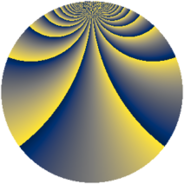# Properties

 Label 2280.2.evLevel $2280$ Weight $2$ Character orbit 2280.ev Rep. character $\chi_{2280}(131,\cdot)$ Character field $\Q(\zeta_{18})$ Dimension $1920$ Sturm bound $960$

# Related objects

## Defining parameters

 Level: $$N$$ $$=$$ $$2280 = 2^{3} \cdot 3 \cdot 5 \cdot 19$$ Weight: $$k$$ $$=$$ $$2$$ Character orbit: $$[\chi]$$ $$=$$ 2280.ev (of order $$18$$ and degree $$6$$) Character conductor: $$\operatorname{cond}(\chi)$$ $$=$$ $$456$$ Character field: $$\Q(\zeta_{18})$$ Sturm bound: $$960$$

## Dimensions

The following table gives the dimensions of various subspaces of $$M_{2}(2280, [\chi])$$.

Total New Old
Modular forms 2928 1920 1008
Cusp forms 2832 1920 912
Eisenstein series 96 0 96

## Trace form

 $$1920q - 6q^{4} + O(q^{10})$$ $$1920q - 6q^{4} + 6q^{10} - 6q^{16} + 24q^{30} + 42q^{34} - 120q^{36} + 54q^{42} - 66q^{48} + 960q^{49} - 48q^{52} - 66q^{54} + 42q^{64} + 60q^{66} + 72q^{70} - 84q^{72} + 48q^{73} + 204q^{76} - 60q^{78} - 24q^{81} + 48q^{88} - 54q^{90} + 24q^{94} - 252q^{96} + O(q^{100})$$

## Decomposition of $$S_{2}^{\mathrm{new}}(2280, [\chi])$$ into newform subspaces

The newforms in this space have not yet been added to the LMFDB.

## Decomposition of $$S_{2}^{\mathrm{old}}(2280, [\chi])$$ into lower level spaces

$$S_{2}^{\mathrm{old}}(2280, [\chi]) \cong$$ $$S_{2}^{\mathrm{new}}(456, [\chi])$$$$^{\oplus 2}$$